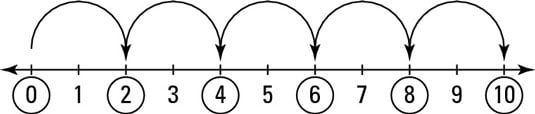Multiplication
Strategies
Multiplication
Division
Strategies
Division
Word Problems
100

What multiplication sentence matches this picture?3 x 5=15

100

Solve using a strategy.

2 x 9

18

100

What division sentence matches this picture?24 ÷ 8=3

100

Solve using a strategy.

14 ÷ 2

7

100

We found 5 boxes of red ornaments for the tree. Each box had 2 ornaments in it. How many ornaments in all?

5 x 2 = 10

200

What multiplication sentence matches this array?4 x 4=16

200

Solve using a strategy.

3 x 7

21

200

What division sentence matches the array?21 ÷ 7=3

200

Solve using a strategy.

24 ÷ 3

8

200

We bought 6 packages of candy canes. Each package has 6 candy canes. How many candy canes in all?

6 x 6 = 36

300

What multiplication sentence matches this repeated addition sentence?

2 + 2 + 2 + 2 + 2 + 2 =12

6 x 2=12

300

Solve using a strategy.

6 x 4

24

300

What division sentence matches the repeated subtraction sentence?

40-8=32

32-8=24

24-8=16

16-8=8

8-8=0

40 ÷ 8=5

300

Solve using a strategy.

35 ÷ 7

5

300

There are 28 gifts under the tree. They are separated equally in 4 bags. How many gifts are in each bag?

7 gifts

400

What multiplication sentence matches the following:

7, 14, 21, 28, 35, 42

6 x 7 =42

400

Solve using a strategy.

6 x 7

42

400

What division sentence matches the following:

2, 4, 6, 8, 10, 12, 14, 16, 18, 20

20 ÷ 2=10

400

Solve using a strategy.

36 ÷ 6

6

400

Our group went caroling at 12 houses. We sang 4 songs at each house. How many songs did we sing in all?

48 songs

500

Identify the multiplication sentence that matches the number line.5 x 2=10

500

Solve using a strategy.

9 x 8

72

500

Identify the division sentence that matches the following:15 ÷ 5=3

500

Solve using a strategy.

56 ÷ 8

500

We decorated 63 cookies and divided them equally onto 7 plates. How many cookies are on each plate?

9 cookies

Click to zoom